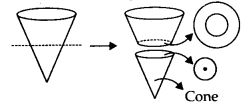# MP Board Class 7th Maths Solutions Chapter 15 Visualising Solid Shapes Ex 15.3

## MP Board Class 7th Maths Solutions Chapter 15 Visualising Solid Shapes Ex 15.3

Question 1.
What cross-sections do you get when you give a
(i) vertical cut
(ii) horizontal cut
to the following solids?
(a) A brick
(b) A round apple
(c) A die
(d) A circular pipe
(e) An ice cream cone
Solution:
(a) Brick
We can give a vertical cut to a brick in the following way.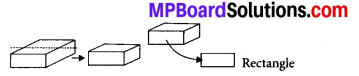We can give a horizontal cut to a brick in the following way.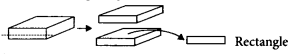(b) A round apple
We can give a vertical cut to a round apple in the following way.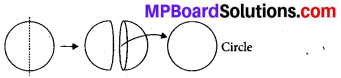We can give a horizontal cut to a round apple in the following way.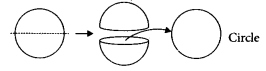(c) A die
We can give a vertical cut to a dice in the following way.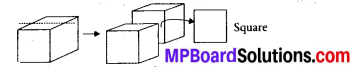We can give a horizontal cut to a dice in the following way.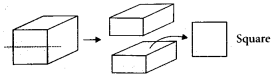(d) A circular pipe
We can give a vertical cut to a circular pipe in the following way.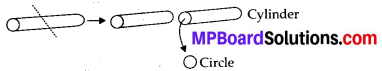We can give a horizontal cut to a circular pipe in the following way.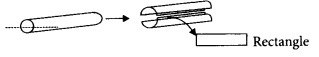(e) An ice cream cone
We can give a vertical cut to an ice cream cone in the following way.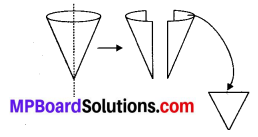We can give a horizontal cut to an ice cream cone in the following way.